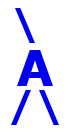## AIRES library reference: ghfcint.

```c
c     Routine directly callable from C/C++ (it could be necessary to
c     append an underscore, "_", to its name depending on the C/C++
c     compiler used).
c
c
function ghfcint(nmax, xmax, x0, lambda)
c
c     Evaluating the complete integral of the Gaisser-Hillas function,
c     i.e.,
c
c            infty
c        I =  INT   Ngh(X) dX
c             X0
c
c     where Ngh(X) is the Gaisser-Hillas function for the profile of
c     charged particles (T. K. Gaisser and A. M. Hillas, in Proc. 15th
c     ICRC (Plovdiv), vol. 8, p. 353 (1977)):
c
c                      /    X - X0   \ [(Xmax-X0)/lambda]
c        Ngh(X) = Nmax | ----------- |                    *
c                      \  Xmax - X0  /
c
c                          /  Xmax - X  \
c                    * exp | ---------- |
c                          \   lambda   /
c
c     for X > X0 (Ngh(X) = 0 for X < X0).
c
c     Written by: S. J. Sciutto, La Plata 2004.
c
c
c     Arguments:
c     =========
c
c     nmax, xmax, x0,
c     lambda.......... (input, double precision) The four parameters of
c                      the Gaisser-Hillas function. Xmax, X0, and
c                      lambda are expressed in g/cm2.
c                      Notice that nmax is used only as a
c                      multiplicative factor.
c
c
c     Return value: (double precision) The value of the integral, or
c     ============  zero in the case of invalid arguments.
c
c
c<--->
```

 These pages are maintained by Sergio J. Sciutto Back to AIRES library index Back to AIRES home